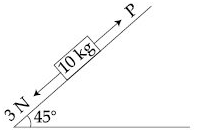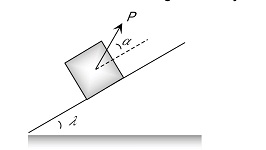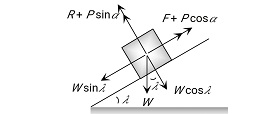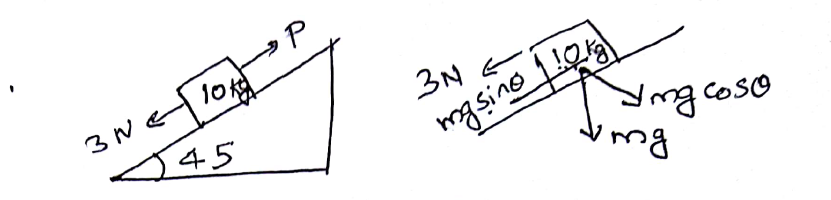# A block of mass 10 Kg is kept on a rough inclined plane as shown in the figure. A force of 3 N is applied on the block. The coefficient of static friction between the plane and the block is 0.6. What should be the minimum value of force P, such that the block doesnot move downward ? (take g =10ms-2)Option 1)32 N  Option 2)18 NOption 3)23 NOption 4)25 N

Minimum Force to avoid sliding of body down on an inclined plane -P = Pulling force

Angle of Inclination

Angle of friction

- wherein

For equilibrium

Use3 + mg sin = P + mg cos

P = 32 N

Option 1)

32 N

Option 2)

18 N

Option 3)

23 N

Option 4)

25 N

Exams
Articles
Questions